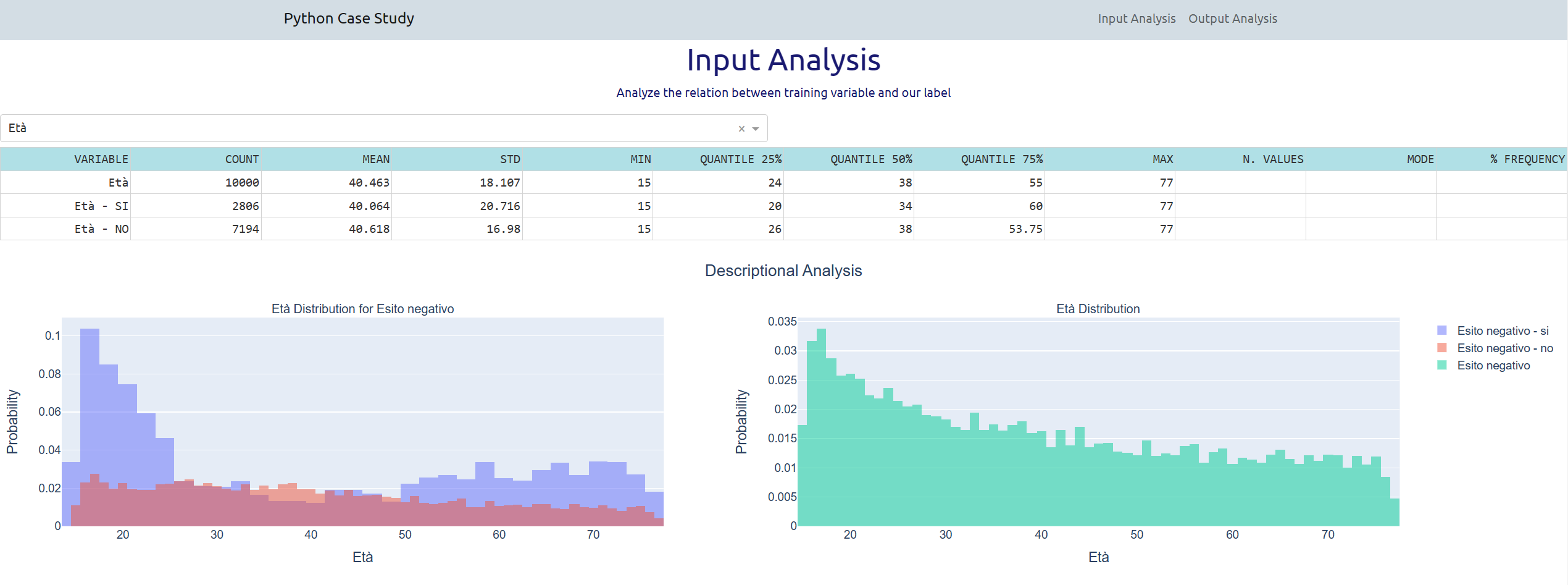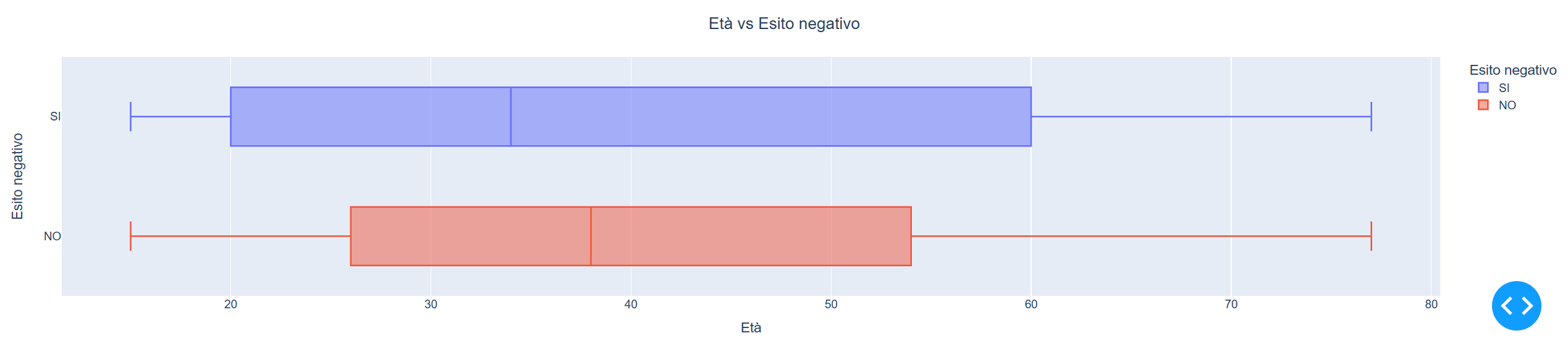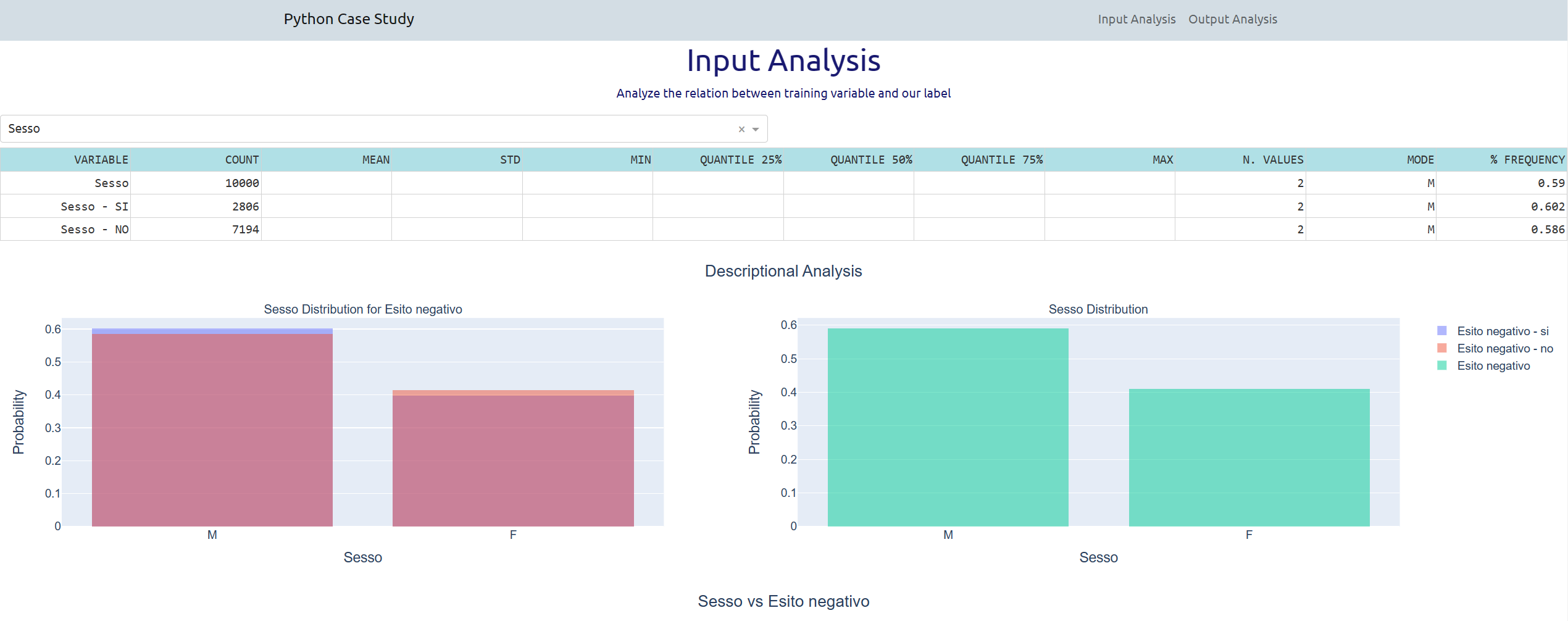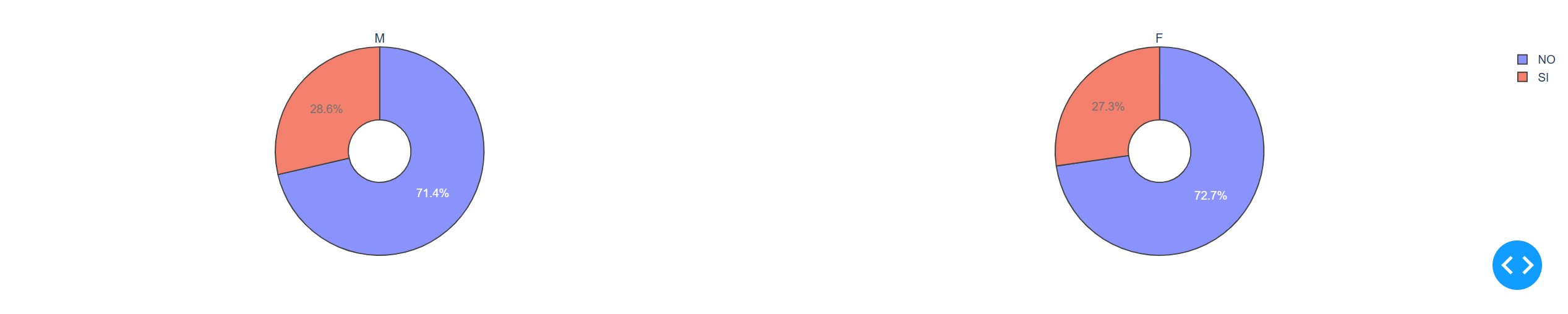﻿

## The Case Study and the Python Dashboard

The Case Study is based on a hypothetical bank credit risk data set.
The data set contains 26 variables collected on 10,000 observations:

1. 13 numerical
2. 13 categorical, including the target variable “Esito Negativo”.

The goal of the Case Study is to analyze the data and to predict the Target Variable “Esito Negativo”.

Click here to see the list of the variables of this Case Study, their possible codes and their English Translation.

This Case Study is developed with Python Jupyter Notebook to load data and apply models, and Plotly Dash to build a dashboard to show the results.

Python is a high-level, general-purpose programming language. Its design philosophy emphasizes code readability in particular with the use of significant indentation to define the end of the statements and the blocks of statements. Python is dynamically-typed and garbage-collected. It supports multiple programming paradigms, including structured (particularly procedural), object-oriented and functional programming. It is open source and full of comprehensive standard library and user defined modules.

The Jupyter Notebook App is a server-client application that allows editing and running notebook documents via a web browser. The Jupyter Notebook App can be executed on a local desktop requiring no internet access (as described in this document) or can be installed on a remote server and accessed through the internet. The notebook document allows to associate Python Code and its results in a graphical user-friendly interface. It's particularly useful to present results of programs interactively modified by the user.

Plotly Dash is a python framework created by Plotly company for creating interactive web applications. With Dash, you don't have to learn HTML, CSS and Javascript in order to create interactive dashboards, you only need python code. It's one ot the most used tool to build dashboard with python code.

The present case study is develped in two phases:

1. a Jupyter notebook to load and control data, and to estimate 4 models

2. a Plotly Dashboard for a comparison among the four predictive models, where the Parallel Tree Boosting gets the best accuracy in competition with Logistical Regression, Random Forest and Decisione Tree models.

Among the models tested to predict the default indicators there are the following:

1. LOG = Logistic Regression Model
2. DT = Decision Tree Model
3. RF = Random Forest Model
4. GB = Parallel Tree Boosting Model

A standard Data Mining process has been developed to load and control the input data, transforming it into actionable dataframes, and to estimate and perform a first comparison.

A Jupyter notebook has been created to:

1. load and control data, input data has been loaded into two dataframes, one for training data and the second one for the test. In particular missing data has beed managed with an alternative specific value, and all the categorical variables has been trasformend into dummy variables.

2. estimate the 4 models, using scikit-learn and xgboost libraries.
The Accuracy is the main error indicator used to evaluate the models, and then Sensitivity, Specificity, F1-Score, AUC and precision indicators. ROC charts have been plotted to manually compare the results.

The picture below describes the standard data mining process steps followed to build data, models and evaluate results.

Click on each picture to enlarge it.

## Second phase: Input Analysis

A interactive dashboard illustrates some statistical indicators and probability distribution in a easy way.

The user can select one of the available variables in the input sample.

If the variable is numerical and continuos, as the selected variable below Età, the user can see:

1. a list of base statistical metrics, Count, Mean, Std, Min, Q25, Q50, Q75, Max in the first row for all the sample, in the following for each categorical value.

2. two histograms, the former on the left with the distribution of negative and positive response of the target variablem, in two colors blue for the negative values (sì, otherwise they are in default) orange for the positive values (no, otherwise they are not in default), and in a red colour when the bars are overlapped.
The latter with all the values in green colour.
The two histograms provide a vision of how the cases are distributed respect the categorical values of the selected variable. In the example below the histograms prove that the most risky categories are the young and older people.

3. a BoxPlot chart with two mippoints, one for the positive value and the last for the negative value.
The BoxPlot chart provides a different perspective, with a synthesis of most of the basic statistics illustrated in the table above the charts, giving an idea of the importance of the two tails and of the concentration of the values around the median.
In particular in this example, with the variable Età, we obtain a different vision of the distribution that hides the concentration of the most risky cases in the young and older people.If the variable is categorical, as the selected variable below Sesso, the user can see:

1. a list of base statistical metrics, useful with categorical variables, such as the Count od the number of observations, the number of distinct values, the Mode, and the percentage frequency of the mode value respect all the observations. In this example 0.59 means that 59% of observation have a Male value (the mode value), which becames 60.2% when the target variable Esineg is equal 'Sì' and 58,6% when it's 'No'.

2. two histograms, the former on the left with the distribution of negative and positive response of the target variablem, in two colors blue for the negative values (sì, otherwise they are in default) orange for the positive values (no, otherwise they are not in default), and in a red colour when the bars are overlapped.
The latter with all the values in green colour.
The two histograms provide a vision of how the cases are distributed respect the categorical values of the selected variable. In the example below the histograms prove that the most risky categories are the male people for a small amount.

3. two pie chart two basic pie charts to show the category with the high percentage of bad default cases.
In particular in this example, the Male people have higher percentage for a slight amount of about 1%.Click on each picture to view it.

## Second phase: Output Analysis

The section about output analysis of the interactive dashboard compares the results of the 4 statistical models.

The user has to select two models to be compared. Like in the example below where the models Parallel Tree Boosting and Logistic Regression are selected.

The table in the right summerizes the main basic indicators and numbers used to evaluate models.

Where:

1. -TruePositive are the cases Positive predicted as Positive by the model.
2. -TrueNegative are the cases Negative predicted as Negative by the model.
3. -FalsePositive are the cases Negative predicted as Positive by the model.
4. -FalseNegative are the cases Positive predicted as Negative by the model.

Then in the DashBoard outputs are shown:

1. a list of error or performance indicators, each one able to identify the best model following several formulas and point of view.
The computed error or performance indicators are:
-Accuracy: the number of correct predition on the total. Otherwise the number of TruePositive+TrueNegative on the total.
-Sensitivity: (or True Positive Rate or Recall) the proportion of positive data points that are correctly considered as positive, with respect to all positive data points. Otherwise the number of TruePositive on the sum of FalseNegative and TruePositive.
-Specificity: (or False Positive Rate) the proportion of negative data points that are correctly considered as negative, with respect to all negative data points. Otherwise the number of TrueNegative on the sum of FalsePositive and TrueNegative.
-Precision: It is the number of correct positive results divided by the number of positive results predicted by the model. Otherwise the number of TruePositive on the sum of FalsePositive and TruePositive.
-F1_score: F1 Score is the Harmonic Mean between precision and recall.
The range for F1 Score is [0, 1]. It tells you how precise your classifier is (how many instances it classifies correctly), as well as how robust it is (it does not miss a significant number of instances). Otherwise is 2 divided by then sum of 1/Precision and 1/Recall.
-AUC: (or Area Under Curve) AUC is the area under the curve of plot False Positive Rate vs True Positive Rate at different points in [0, 1]. AUC has a range of [0, 1]. AUC is one of the most widely used metrics for evaluation, used for binary classification problem. AUC of a classifier is equal to the probability that the classifier will rank a randomly chosen positive case higher than a negative one.

For all these indicators, the greater the value, the better is the performance of our model.

2. a classical ROC curve, that allows you to compared the results of the two model respect a casual one represented by the crossed dashed line.

3. two confusion matrix, one for each model.
The confusion matrix allows you to evaluate graphically a model in term of number or percentage of cases falling in the four sector, where tipically the most important sector is the one in the upper right with the true positive events detected by the model, the default of the client in this case. The second sector in importance if the true negative events.

Between the two selected models in the example above, the Parallel Tree Boosting is always better than the Logistic Regression, easily to imagine considering that the Boosting has more parameters to fit the model and requires more time to detect the best Tree sub model.

Click on the video below to see the dashboard in action for the visualization of the values of each column of a selected data set and to see a quick view of the component data flow that generates and controls the dashboard.

Click on each picture to view it.